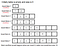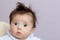# The Amortized time complexity of increasing Array size

Feel free to checkout this blog to see increasing array size in action.

Firstly what is Amortized time complexity?

According to Wikipedia: In computer science, amortized analysis is a method for analyzing a given algorithm’s complexity, or how much of a resource, especially time or memory, it takes to execute. The motivation for amortized analysis is that looking at the worst-case run time per operation, rather than per algorithm, can be too pessimistic.

In simple words: Instead of calculating time complexity of an algorithm based on its worst case operation, we calculate amortized time complexity of an algorithm by calculating average time taken by all operations.

## So how would we increase the size of an array?

Basic Approach: Whenever we need more space, increase it to the new required size. Example:
If the current array size is 4 and we want an array of size 5. Then we will create a new array of size 5 and copy all 4 elements in the new array.
Time Complexity will be O(n) //Copying old elements into a new array.
Amortized Time Complexity will also be O(n), as every time we need to copy all elements

Better Approach: Let's understand another algorithm for increasing the array size: Whenever we will face an overflow we will increase size of the array to the double of original size.credit: geeksforgeeks.org

Now, if you see when we are trying to insert 5, we have created a new array of size 8 and copied the previous 4 elements and then inserted 5.

The Time Complexity of this algorithm is also O(n) where n is the number of elements in the array. As, In the worst case(at the time of inserting 5) we are copying all the 4 elements in the new array.

Let’s consider that each copy of an element will cost us 1 CPU cycle. When we are calculating the time complexity we see the worst case of the algorithm, it will be when we are trying to insert the 5th element as we are increasing array size to 8 and copying all the elements in the new array. This will cost us 4 CPU cycles. So to perform the operation of inserting 5 in the array we will require 4 CPU cycles to perform that operation.

## What is the Amortized Time complexity of the above algorithm?Credit: mnn.com

For a second, let's stop looking at the worst-case scenario. What if I tell you that we can perform any operation(irrespective of the current size of the array) just by 2 CPU cycle.
That’s right, We will give 2 CPU cycles to each operation in this algorithm. Now, let’s see how we will be able to complete all operations.

Let’s dry run the algorithm from 2nd operation where we have just increased the size of the array from 2 to 4 and trying to insert 3. As we promised we will give 2 CPU cycles to each operation. After inserting 3 we haven’t copied anything, so element 3 has 2 CPU cycles in his pocket💰💰. Now when we put 4, again we haven’t copied anything so both 3 and 4 have 2 CPU cycles in their pockets💰💰💰💰.

The element 5 came with 2 CPU cycle and now we want to copy the whole array in a new array of size 8. As both 3 and 4 have 2–2 CPU cycles, they will pay for themselves as well as for 1 and 2 element(other half of the array). The total CPU cycles array had was 4 which is equal to CPU cycles need to copy all element into the new array.
So the operation was completed and now new element 5 will come with his 2 CPU cycle in his pocket💰💰, similarly 6,7,8 elements. When the 9th element will come we need to copy the whole array. Again total CPU cycles array has is 8(2 of 5th+2 of 6th+2 of 7th+2 of 8th) and we will be needing 8 as we need to copy whole array(8 elements). Again the operation will be completed.

## So in this way always half of the array will have 2 CPU cycles and will pay for the whole array in the worst-case scenarios where we need to copy the whole array.

In this way, the worst case time complexity is still O(n) which is equal to basic approach, but Amortized time complexity went straight to O(2){as we just need to give 2 CPU cycles for each operation} from O(n) of basic approach.